The materials can be classified by the energy gap between their valence band and the conduction band. The valence band is the band consist...

The materials can be classified by the energy gap between their valence band and the conduction band. The valence band is the band consisting of the valence electron, and the conduction band remains empty. Conduction takes place when an electron jumps from valence band to conduction band and the gap between these two bands is forbidden energy gap. Wider the gap between the valence and conduction bands, higher the energy it requires for shifting an electron from valence band to the conduction band. In the case of conductors, this energy gap is absent or in other words conduction band, and valence band overlaps each other. Thus, electron requires minimum energy to jump from valence band. The typical examples of conductors are Silver, Copper, and Aluminium. In insulators, this gap is vast. Therefore, it requires a significant amount of energy to shift an electron from valence to conduction band. Thus, insulators are poor conductors of electricity. Mica and Ceramic are the well-known examples of insulation material.
Semiconductors, on the other hand, have an energy gap which is in between that of conductors and insulators. This gap is typically more or less 1 eV, and thus, one electron requires energy more than conductors but less than insulators for shifting valence band to conduction band. At low temperature there are very less number of electrons in conduction band in a semiconductor crystal but when the temperature is increased more and more electrons get sufficient energy to migrate from valence band to conduction band. Because of that, they don’t conduct electricity at low temperature but as the temperature increases the conductivity increases. The most typical examples of the semiconductors are silicon and germanium.

### Definition of Semiconductor

Thus, the definition of semiconductor can be as follows.

The materials that are neither conductor nor insulator with energy gap of about 1 eV (electron volt) are called semiconductors.

Most common type of materials that are commertially used as semiconductors are germanium (Ge) and silicon (Si) because of their property to withstand high temperature. That means there will be no signicant change in energy gap with changing temperature. The relation between energy gap and absolute temperature for Si and Ge are given as,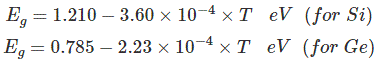Where, T = absolute temperature in oK
Assuming room temperature to be 300oK,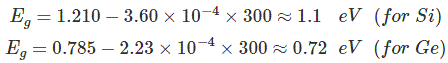At room temperature resistivity of semiconductor is in between insulators and conductors. Semiconductors show negative temperature coefficient of resistivity that means its resistance decreases with increase in temperature. Both Si and Ge are elements of IV group i.e. both elements have 4 valence electrons. Both form the covalent bond with the neighbouring atom. At absolute zero temperature both behave as insulator i.e. the valence band is full while conduction band is empty but as the temperature is raised more and more covalent bonds break and electrons are set free and jump to the conduction band.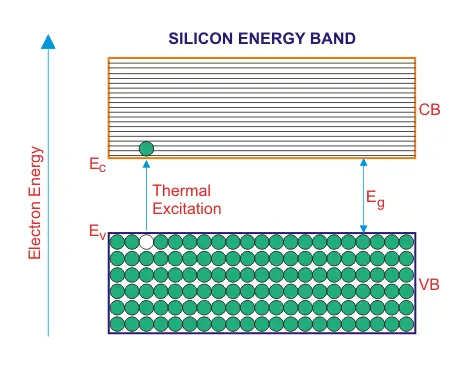In the above energy band diagrams of a semiconductor. CB is the conduction band and VB is the valence band. At 0°K, the VB is full with all the valence electrons.

### Intrinsic Semiconductors

As per theory of semiconductor, semiconductor in its pure form is called as intrinsic semiconductor. In pure semiconductor number of electrons (n) is equal to number of holes (p) and thus conductivity is very low as valence electrons are covalent bonded. In this case we write n = p = ni, where ni is called the intrinsic concentration. It can be shown that ni can be written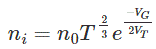Where, n0 is a constant, T is the absolute temperature, VG is the semiconductor band gap voltage, and VT is the thermal voltage.

The thermal voltage is related to the temperature by VT = kT/q
Where, k is the Boltzmann constant (k = 1.381 × 10 − 23 J/K).
In intrinsic semiconductors conductivity (σ) is determined by both electrons (σe) and holes (σh) and depends on the carrier density.
σe = neμe, σh = peμh
Conductivity,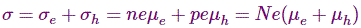Where n, p = numbers of electrons and holes respectively.
μh, μe = mobility of free holes and electrons respectively
N = n = p e = charge on carrier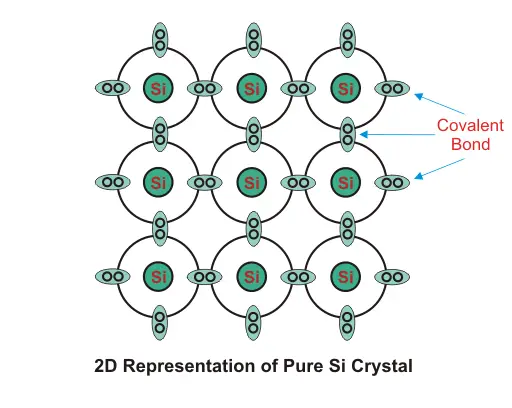### Extrinsic Semiconductors

As per theory of semiconductor, impure semiconductors are called extrinsic semiconductors. Extrinsic semiconductor is formed by adding a small amount of impurity. Depending on the type of impurity added we have two types of semiconductors: N - type and P-type semiconductors. In 100 million parts of semiconductor one part of impurity is added.

### N type Semiconductor

In this type of semiconductor majority carriers are electrons and minority carriers are holes. N - type semiconductor is formed by adding pentavalent (five valence electrons) impurity in pure semiconductor crystal, e.g. P. As, Sb.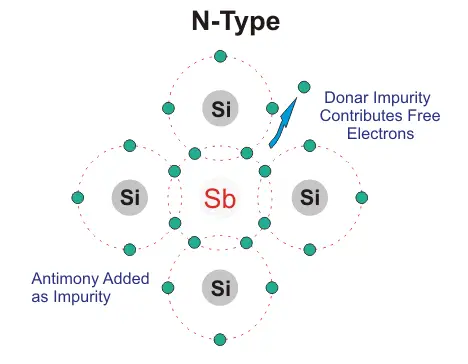Four of the five valence electron of pentavalent impurity forms covalent bond with Si atom and the remaining electron is free to move anywhere within the crystal. Pentavalent impurity donates electron to Si that’s why N- type impurity atoms are known as donor atoms. This enhances the conductivity of pure Si. Majority carriers are electrons therefore conductivitry is due to these electrons only and is given by,
σ = neμe

### P-type Semiconductors

In this type of semiconductor majority carriers are holes and minority carriers are electrons. P- type semiconductor is formed by adding trivalent ( three valence electrons) impurity in pure semiconductor crystal, e.g. B, Al Ba.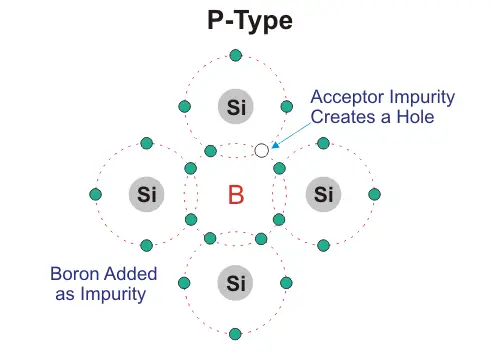Three of the four valence electron of tetravalent impurity forms covalent bond with Si atom. This leaves an empty space which is referred to as hole. When temperature is raised electron from another covalent bond jumps to fill this empty space. This leaves a hole behind. In this way conduction takes place. P- type impurity accepts electron and is called acceptor atom. Majority carriers are holes and therefore conductivity is due to these holes only and is given by,
σ= neμhName

BASIC ELECTRICAL,12,BATTERIES,4,CIRCUIT THEORIES,9,CONTROL SYSTEMS,3,DC MOTOR,1,DIGITAL ELECTRONICS,1,DISTRIBUTED GENERATION,2,DISTRIBUTION,6,ELECTRICAL DRIVES,1,ELECTRICAL LAWS,8,ELECTRONICS DEVICES,2,General,7,GENERATION,3,GENERATOR,1,HIGH VOLTAGE,4,ILLUMINATION,1,INDUCTION MOTOR,7,MATERIALS,1,MEASUREMENT,1,MOTOR,1,POWER ELECTRONICS,2,PROJECTS ON INDUCTION MOTOR,1,PROTECTION,1,SMART GRID,3,SWITCHGEAR,4,SYNCHRONOUS MOTOR,1,TRANSFORMER,6,TRANSMISSION,4,
ltr
item
Electrical for Us: Theory of Semiconductor
Theory of Semiconductor
http://www.electrical4u.com/images/march16/1462725463.GIF
Electrical for Us
http://www.sanjaysah.com.np/2016/11/theory-of-semiconductor.html
http://www.sanjaysah.com.np/
http://www.sanjaysah.com.np/
http://www.sanjaysah.com.np/2016/11/theory-of-semiconductor.html
true
3851448774078769448
UTF-8